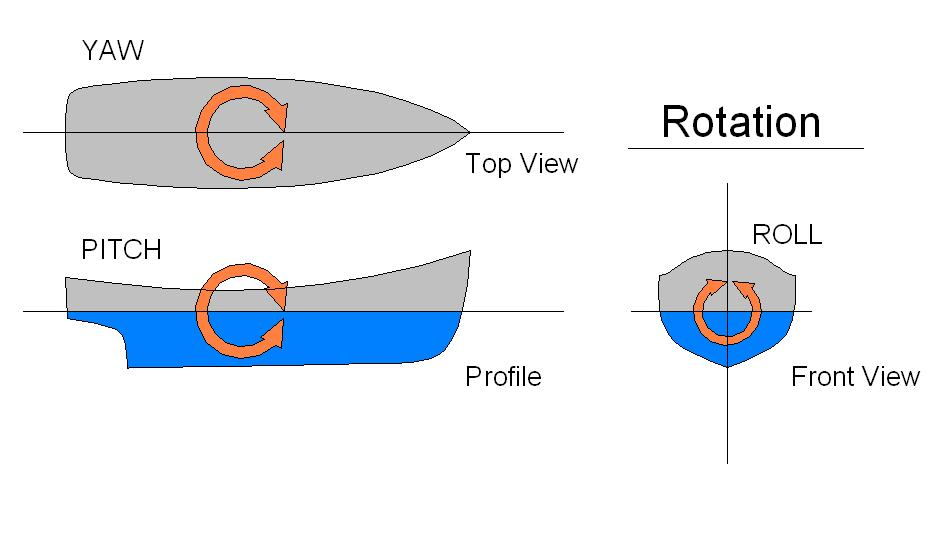# How To Convert Euler Angles to Quaternions Using PythonGiven Euler angles of the following form….

• Rotation about the x axis = roll angle = α
• Rotation about the y-axis = pitch angle = β
• Rotation about the z-axis = yaw angle = γ

…how do we convert this into a quaternion of the form  (x, y, z, w) where w is the scalar (real) part and x, y, and z are the vector parts?

Doing this operation is important because ROS2 (and ROS) uses quaternions as the default representation for the orientation of a robot in 3D space.

Here is the Python code:

```#! /usr/bin/env python3

# This program converts Euler angles to a quaternion.

import numpy as np # Scientific computing library for Python

def get_quaternion_from_euler(roll, pitch, yaw):
"""
Convert an Euler angle to a quaternion.

Input
:param roll: The roll (rotation around x-axis) angle in radians.
:param pitch: The pitch (rotation around y-axis) angle in radians.
:param yaw: The yaw (rotation around z-axis) angle in radians.

Output
:return qx, qy, qz, qw: The orientation in quaternion [x,y,z,w] format
"""
qx = np.sin(roll/2) * np.cos(pitch/2) * np.cos(yaw/2) - np.cos(roll/2) * np.sin(pitch/2) * np.sin(yaw/2)
qy = np.cos(roll/2) * np.sin(pitch/2) * np.cos(yaw/2) + np.sin(roll/2) * np.cos(pitch/2) * np.sin(yaw/2)
qz = np.cos(roll/2) * np.cos(pitch/2) * np.sin(yaw/2) - np.sin(roll/2) * np.sin(pitch/2) * np.cos(yaw/2)
qw = np.cos(roll/2) * np.cos(pitch/2) * np.cos(yaw/2) + np.sin(roll/2) * np.sin(pitch/2) * np.sin(yaw/2)

return [qx, qy, qz, qw]
```

# Example

Suppose a robot is on a flat surface. It has the following Euler angles:

Euler Angle (roll, pitch, yaw) = (0.0, 0.0, π/2) = (0.0, 0.0, 1.5708)

What is the robot’s orientation in quaternion format (x, y, z, w)?

The program shows that the quaternion is:

Quaternion [x,y,z,w] = [0, 0, 0.7071, 0.7071]

And that’s all there is to it folks. That’s how you convert a Euler angles into a quaternion.